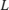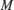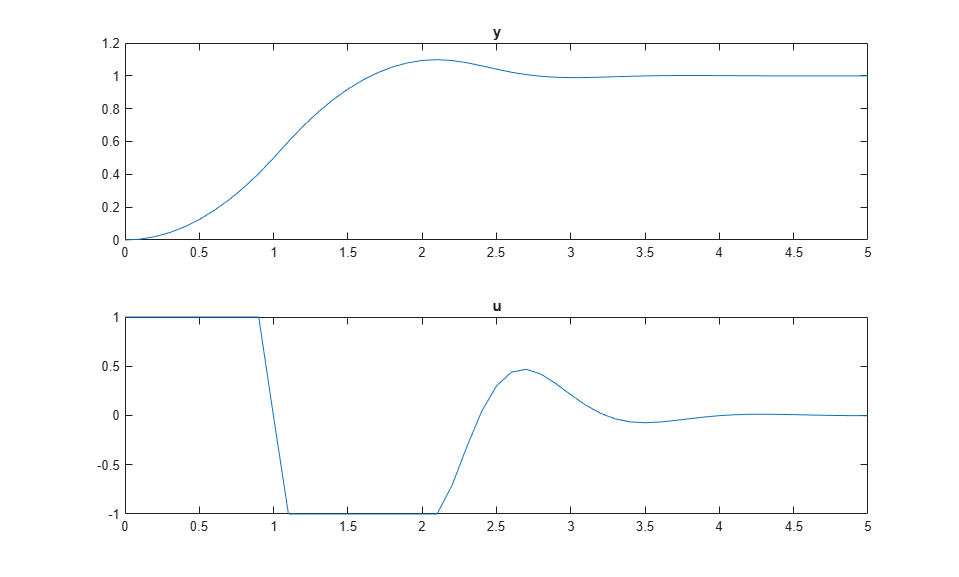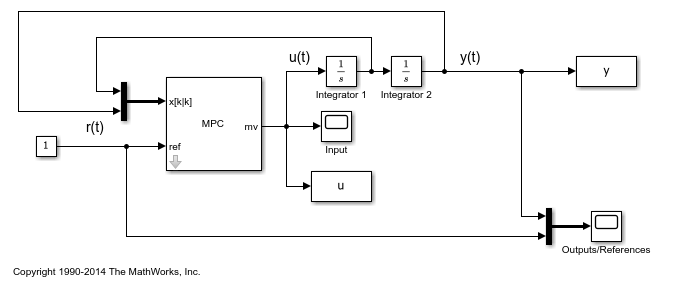# Custom State Estimation

Model Predictive Control Toolbox™ software allows you to override the default controller state estimation method.

To do so, you can use the following methods:

• You can override the default Kalman gains,and, using the `setEstimator` function. To obtain the default values from the controller use `getEstimator`. These commands assume that the columns ofandare in the engineering units of the measured plant outputs. Internally, the software converts them to dimensionless form.

• You can use the custom estimation option, which skips all Kalman gain calculations within the controller. When the controller operates, at each control interval you must use an external procedure to estimate the controller states and provide these state estimates to the controller.

Custom state estimation is not supported in MPC Designer. For more information see Controller State Estimation and Implement Custom State Estimator Equivalent to Built-In Kalman Filter.

### Define Plant Model

Consider the case of a double integrator plant for which all of the plant states are measurable. In such a case, you can provide the measured states to the MPC controller rather than have the controller estimate the states.

The linear open-loop plant model is a double integrator.

```plant = tf(1,[1 0 0]); ```

### Design MPC Controller

Create the controller object with a sample time of `0.1` seconds, a prediction horizon of `10` steps, and control horizon of `3` steps.

```Ts=0.1; mpcobj = mpc(plant,Ts,10,3); ```
```-->"Weights.ManipulatedVariables" is empty. Assuming default 0.00000. -->"Weights.ManipulatedVariablesRate" is empty. Assuming default 0.10000. -->"Weights.OutputVariables" is empty. Assuming default 1.00000. ```

Specify actuator saturation limits as manipulated variable constraints.

```mpcobj.MV = struct('Min',-1,'Max',1); ```

Configure the controller to use custom state estimation.

```setEstimator(mpcobj,'custom'); ```

### Simulate Controller

Initialize variables to store the closed-loop responses.

```Tf = round(5/Ts); YY = zeros(Tf,1); UU = zeros(Tf,1); ```

Prepare the plant used in the simulation by converting it to a discrete-time model and setting the initial state.

```sys = c2d(ss(plant),Ts); xsys = [0;0]; ```

Get an handle to the `mpcstate` object that is used to store the controller states.

```xmpc = mpcstate(mpcobj); ```
```-->Converting the "Model.Plant" property to state-space. -->Converting model to discrete time. Assuming no disturbance added to measured output #1. -->"Model.Noise" is empty. Assuming white noise on each measured output. ```

Iteratively simulate the closed-loop response using the `mpcmove` function.

For each simulation step:

• Obtain the plant output, `y`, from the current state.

• Store the plant output.

• Set the plant state in the `mpcstate` object to the current measured state values, `xsys`, using the handle `xmpc`. For plants in which the state is not measurable, a state estimation must be provided by an observer. When using the built-in estimator, this is not needed.

• Compute the MPC control action, `u`, passing in the `mpcstate` object and the output reference, `1`.

• Store the control signal.

• Update the plant state.

```for t = 0:Tf y = sys.C*xsys; % plant equations: output YY(t+1) = y; xmpc.Plant = xsys; % state estimation u = mpcmove(mpcobj,xmpc,[],1); % y is not needed UU(t+1) = u; xsys = sys.A*xsys + sys.B*u; % plant equations: next state end ```

Plot the simulation results.

```figure subplot(2,1,1) plot(0:Ts:5,YY) title('y') subplot(2,1,2) plot(0:Ts:5,UU) title('u') ```Simulate closed-loop control of the linear plant model in Simulink. For this model, the controller `mpcobj` is specified in the MPC Controller block.

```mdl = 'mpc_customestimation'; open_system(mdl) sim(mdl) ```The closed-loop responses for the MATLAB and Simulink simulations are identical.

```fprintf('\nDifference between simulations in MATLAB and Simulink is %g\n',norm(UU-u)); ```
```Difference between simulations in MATLAB and Simulink is 5.39874e-14 ```

```bdclose(mdl) ```